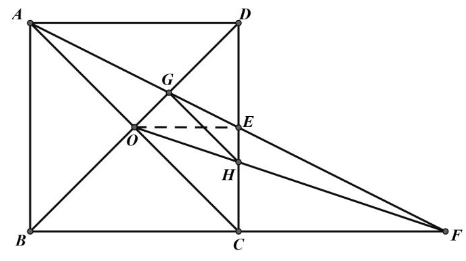【答案】

\begin{aligned} & \Leftrightarrow O E / / B C, A D=C F \\ & \Leftrightarrow \frac{E H}{C H}=\frac{O E}{F C}=\frac{1}{2} \\ & \Leftrightarrow \frac{D H}{C H}=\frac{2}{1}=\frac{A D}{F B}=\frac{D G}{B G} \\ & \Leftrightarrow \frac{D H}{C H}=\frac{D G}{G O}(O \text { 是 } B D \text { 中点) } \\ & \Leftrightarrow G H / / O C \\ & \Leftrightarrow G H=G D \\ & \Leftrightarrow O G+G H=O D=A O \end{aligned}
(2) 取 $B C$ 中点 $K$, 则由 Rt $\triangle F C H$
$\sim$ Rt $\triangle F K O$
$\frac{C H}{K O}=\frac{C F}{K F}$, 即 $\frac{C H}{\frac{1}{2}}=\frac{C F}{C F+\frac{1}{2}}$, 展开得 $C F \cdot C H=\frac{C F-C H}{2}$